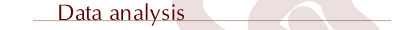search  | feedbackthriving   organisationsgovernanceplanningevaluationquality   improvementevidence   based practicerisk managementwriting policy   & organisational   manualsquestionnairesdata analysissocial capitalfacilitationtraining workshopsideas & articlesabout usdisclaimer | copyrightManagement   Alternatives Pty Ltd   ABN 23 050 334 435Contents | 1. Introduction | 2. Qualitative | 3. Quantitative | 4. Software tools | 5. Resources

3. Quantitative analysis: Questions to ask

There are different kinds of questions it can be useful to ask. To answer these questions will require numerical tools. In the following examples imagine we have survey data from a group of 300 clients.

The survey data from these clients includes:

• Data about themselves, such as age, address, family type
• Data about services provided (what services, how much, how often)
• Data about the service process( how long did I wait on the waiting list)
• Data about views about the quality of services (eg the service is timely, the staff are courteous)
• Data about the benefits of services (I am feeling more confident in parenting in stressful situations).

In the client survey data above the variables are the questions you ask, for example: What is your age? Age is a variable. Is the service timely? The response is a variable.

Examples of questions about variables are:

• What is the average age of clients?
• What is the age range of clients?
• What is the age at which half the clients are that age or older and half are that age or younger?
• What percentage of clients are from a specific geographic area?
• What percentage of clients received a particular type of service?
• How many clients were more confident in parenting in stressful situations?

Answering these questions requires the use of numerical tools, for example:

• Mean
• Median
• Mode
• Range
• Inter-quartile range
• Frequency distribution

Questions about the relationships between one variable with another

Questions can be about how one variable relates with another. For example:

• What is the relationship between the age and gender of clients?
• What is the relationship between the age of clients and whether the clients thought the service was timely?
• What is the relationships between the timeliness of the service and whether the clients were more confident in parenting in stressful situations?

Answering these questions requires the use of numerical tools, for example:

• Cross-tabulations
• Correlations
• Analysis of variance

Questions about the relationships between several variables with another

Questions can be about how several variables relates with each other. For example:

• What is the relationship between the demographic variables and the outcome variables?
• What is the relationship between the quality of service variables and the outcome variables?

Answering these questions requires the use of numerical tools, for example:

• Multi-way frequency analysis
• Multiple correlation

Questions about differences between groups

Questions can be about the differences between groups of clients. For example:

• How are male and female clients similar? different?
• How are older clients and young clients similar? different?

Answering these questions requires the use of numerical tools, for example:

• Analysis of variance
• Multivariate analysis of variance
• Profile analysis.

Question about types or clusters of clients

Questions can be about how to groups clients together into types of clients, or clusters of clients. For example:

• Can we cluster clients into four groups so that each of the groups has a different profile (in terms of demographics and services provided) that makes sense to use in terms of service practice?

Answering these questions requires the use of numerical tools, for example:

• Cluster analysis

Questions about underlying structure in the data

Questions can be about the underlying structure in the data. For example, we may have asked 8 questions about the perceived quality of services. We can ask?

• How are these eight questions related to each other?
• Are there some underlying factors?

Answering these questions requires the use of numerical tools, for example:

• Factor analysis
• Principal components analysis
• Structural equation modeling